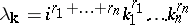# Hyperbolic cross

A summation domain of multiple Fourier series (cf. also Partial Fourier sum). Let $f ( x )$ be an integrable periodic function of $n$ variables defined on $\mathbf{T} ^ { n }$, $\mathbf{T} = ( - \pi , \pi ]$. It has an expansion as a Fourier series, $\sum _ { \mathbf{k} } c_{ \mathbf{k} } e ^ { i \mathbf{kx} }$, $\mathbf{k} = ( k _ { 1 } , \dots , k _ { n } )$, ${\bf x} = ( x _ { 1 } , \ldots , x _ { n } )$, $\mathbf{k} \cdot \mathbf{x} = k _ { 1 } x _ { 1 } + \ldots + k _ { n } x _ { n }$. Unlike in the one-dimensional case, there is no natural ordering of the Fourier coefficients, so the choice of the order of summation is of great importance.
Let $\mathbf{r} = ( r _ { 1 } , \dots , r _ { n } ) \in \mathbf{R} ^ { n }$ with all coordinates positive, $r_j > 0$. Consider the differential operatorwith periodic boundary conditions on $\mathbf{T} ^ { n }$. Then the eigenvalues (cf. Eigen value) of $D ^ { \mathbf{r} }$ are, while the corresponding eigenfunctions are $e ^ { i \mathbf k . \mathbf x }$. The partial sums of the Fourier series corresponding to the eigenfunctions with all eigenvalues $| \lambda _ { \mathbf{k} } | \leq N$ are called hyperbolic partial Fourier sums of order $N$ (or hyperbolic crosses). This approach, in which the method of summation of the Fourier series is defined by the differential operator, is due to K. Babenko [a1], who applied it to various problems in approximation theory (e.g., Kolmogorov widths, $\varepsilon$-entropy, etc.). Subsequently the hyperbolic cross itself became the object of study in connection with Lebesgue constants, the Bernshtein inequality, etc. Also, this approach initiated a detailed study and applications of spaces of functions with bounded mixed derivative (in $L _ { p }$).